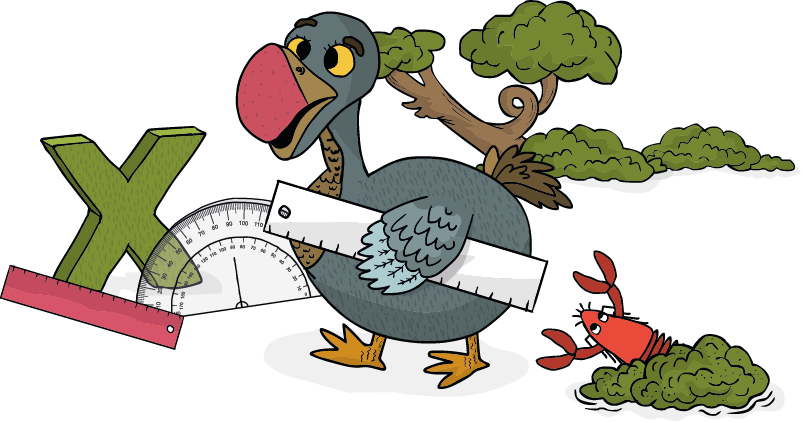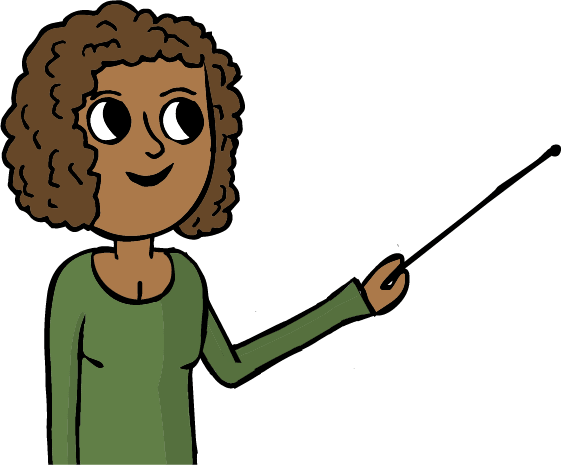# Dodo Does Math aligns to the 2nd-4th grade Common Core Standards and offers students a memorable way to practice math through computer coding.

free trial, credit card not required### MATH AND CODING FOR KIDS

In each exercise, students need to correctly use 2nd-4th grade math concepts, as well as basic code.

Since student solutions are shown on the screen in real-time, visual learners can effectively grasp the math curriculum. Each challenge also incorporates CoffeeScript code, so students utilize a real-world coding language that is as useful as JavaScript but as friendly as Python.

free trial, credit card not required

## CURRICULUM

Measuring

20 Challenges

#### COURSE LENGTH

Classroom lessons plans: 3

#### MATH CONCEPTS

Angles Measuring, Use of Protractor

20 Challenges

#### COURSE LENGTH

Classroom lessons plans: 3

#### MATH CONCEPTS

Commutative & Distributive
Properties, Skip Counting,  Multiplication

20 Challenges

#### COURSE LENGTH

Classroom lessons plans: 3

### EMBARK ON A MATHEMATICAL ADVENTURE

#### INTEGRATED LEARNING PROVIDES BETTER MATH SKILLS

The saying don’t put all your eggs in one basket was practically made for teachers. Educators teach different subjects so that students are exposed to as many fields as possible and have enough career choices to fall back on. Through introducing Computer Science, teachers expand the opportunities students are presented with in school.

If you don’t have enough time to teach computer programming in school, you can add an element of computer programming while teaching math. Dodo Does Math combines two important fields – math and computer programming – so that your students develop better computational and mathematical skills.

#### PRACTICE DISTANCES, ANGLES AND MULTIPLICATION

Put your students’ knowledge of distances, angles and multiplication to the test with 60 interactive challenges. In Dodo Does Math: Distances, Students will practice distances with a platform that includes adding, subtracting and measuring – all using a life-like ruler. Students will also get to use a digital protractor in Dodo Does Math: Angles.

In this course, students will write real code as they add angles and distances together to help get the dodo to the eggs. In Dodo Does Math: Multiplication, students will improve their knowledge of the times table as they calculate the amount of lobsters to throw across the ocean so the dodo can get to her eggs.

SELF-GUIDED LEARNING PLATFORM

With guidance throughout the way, practicing math and code has never been easier.

Since learning programming at a young age helps students gain a lifetime of opportunities, the more intuitive the platform, the better.

Assign Dodo Does Math as extra practice. Students can independently continue practicing after-school without an issue since an AI system runs in the background and guides students when they make a mistake.

## STANDARDS ALIGNMENT### COMMON CORE STATE STANDARDS

• CCSS.MATH.CONTENT.2.OA.B.2
• CCSS.MATH.CONTENT.2.NBT.A.2/.B.5 /.B.7
• CCSS.MATH.CONTENT.2.MD.A. 1
• CCSS.MATH.CONTENT.3.OA.B.5/C.7​
• CCSS.MATH.CONTENT. 3.NBT.A.2
• CCSS.MATH.CONTENT.4.MD./C.7

### PRACTICED MATH CONCEPTS

Through providing Dodo Does Math, your students will practice the following mathematical concepts

• Distances